# How do you write a fraction(a/b) in LaTeX?

Fraction is divided into two parts, one is numerator and the other is denominator. According to the structure of the fraction, \frac command has been created in Latex, in which you can pass two arguments.

\frac{numerator}{denominator}
\documentclass{article}
\begin{document}
$\frac{a}{b}, \frac{c}{d}$
$\hat{a} = \frac{\vec{a}}{|\vec{a}|}$
$S_n = \sum_{i=1}^n \frac{r_i}{k}$
\end{document}

Output :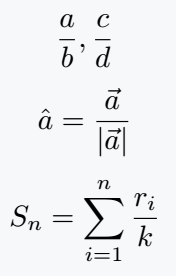You will see the use of more different commands below. And each command will have the option to pass two arguments.

## Fraction Slash

You can use fraction slash in direct code with the help of a keyboard. And in the following latex code, you can use both \divslash and \fracslash commands to complete the task.

\documentclass{article}
\usepackage{fdsymbol, stix}
\begin{document}
$a/b$
$c \divslash d$
$m \fracslash n$
\end{document}

Output :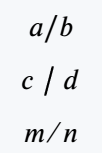## Use diagonal fraction in text mode

In the case of diagonal fraction, the denominator and the numerator are located along the diagonal which is divided by the Fraction Slash. Let’s look at the use of two commands for this.

\documentclass{article}
\usepackage{nicefrac, xfrac}
\begin{document}
$\nicefrac{a}{b}$
$\sfrac{c}{d}$
$i)\; \textrm{What is the multiplication of \;\nicefrac{a}{b} and \;\sfrac{c}{d} ?}$
\end{document}

Output :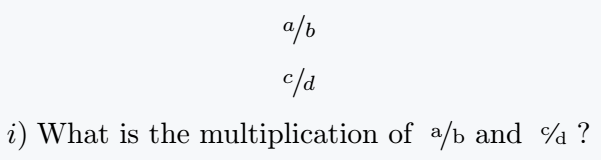When you use fraction in text mode, you should not use a large fraction. But instead, use diagonal fraction or slash.

\documentclass{article}
\usepackage{nicefrac, xfrac}
\begin{document}
i) What is the multiplication of \sfrac{a}{b} and \sfrac{c}{d} ?\\
ii) How do you know that \nicefrac{50}{100} = \nicefrac{1}{2}?\\
iii) Take \sfrac{1}{2} cup of suger,...
\end{document}

Output :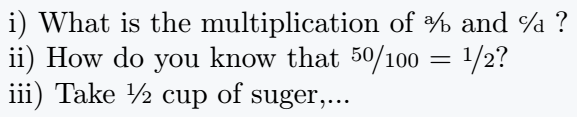## Fraction over fraction in LaTeX

When you implement a nested fraction in a document, you will encounter some morphological problems.

\documentclass{article}
\begin{document}
$\frac{1+\frac{a}{b}}{1+\frac{1}{1+\frac{1}{a}}}$
$\frac{\frac{a}{k}+ \frac{b}{k}}{\frac{c}{k}+ \frac{d}{\frac{1}{k}}}$
\end{document}

Output :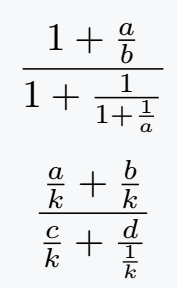And you will see that the size of each part of the above output expression is not equal. For the solution of this problem, you have to use \cfrac instead of \frac Command.

\documentclass{article}
\usepackage{amsmath}
\begin{document}
$\cfrac{1+\cfrac{a}{b}}{1+\cfrac{1}{1+\cfrac{1}{a}}}$
$\cfrac{\cfrac{a}{k}+ \cfrac{b}{k}}{\cfrac{c}{k}+ \cfrac{d}{\cfrac{1}{k}}}$
\end{document}

Output :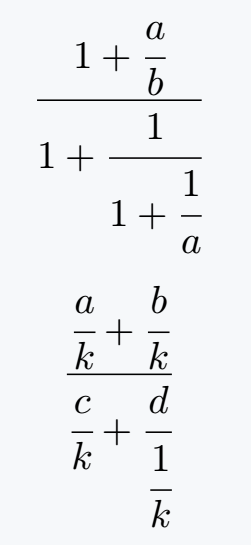Of course, amsmath must be inserted into the document before implementing the \cfrac command. And below are some more examples of a continuous fraction.

And you will see that the size of each part of the above output expression is not equal. For the solution of this problem, you have to use \cfrac instead of \frac Command.

\documentclass{article}
\usepackage{amsmath}
\begin{document}
$a_0 + \cfrac{1}{a_1 + \cfrac{1}{a_2 + \cfrac{1}{\ddots + \cfrac{1}{a_n}}}}$
$1 + \cfrac{1}{1 + \cfrac{2}{1 + \cfrac{1}{1 + \cfrac{2}{1 + \cfrac{1}{1 + \cfrac{2}{\ddots \infty}}}}}}$
\end{document}

Output :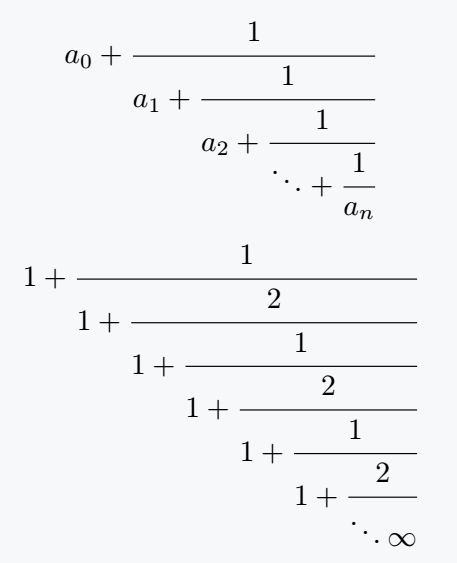## Fraction without line

You will see many expressions whose two elements will exist as numerator and denominator. But, there will be no horizontal line in between. Which we call fraction without a line.

\documentclass{article}
\usepackage{amsmath}
\begin{document}
$\frac{a}{b}, \substack{a\\b}$
$\frac{a}{b}, \genfrac{}{}{0pt}{}{a}{b}$
\end{document}

Output :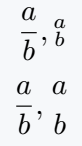I will not recommend using \substack command in the document. However, although the syntax of the \genfrac command is complex, this is the best practice to use.

\documentclass{article}
\usepackage{amsmath}
\newcommand{\fracx}{\genfrac{\{}{\}}{0pt}{}}
\newcommand{\fracy}{\genfrac{[}{]}{0pt}{}{#1}{#2}}
\begin{document}
$\genfrac{}{}{0pt}{}{a}{b}, \genfrac{(}{)}{0pt}{}{a}{b}$
%You can also create and use your own commands.
$\fracx{a}{b}$
$\fracy{c}{d}$
\end{document}

Output :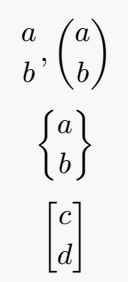#### Md Jidan Mondal

LaTeX expert with over 10 years of experience in document preparation and typesetting. Specializes in creating professional documents, reports, and presentations using LaTeX.